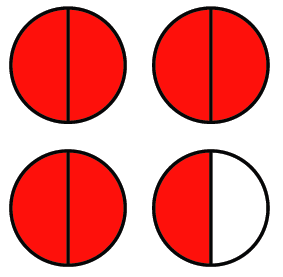### Home > CC1MN > Chapter 6 > Lesson 6.1.3 > Problem6-24

6-24.

Write each of the mixed numbers below as a fraction greater than one, and write each of the fractions greater than one as a mixed number. Include a diagram to explain each answer.

Refer to the Math Notes box at the bottom of the page for help.

1. $4 \frac { 1 } { 3 }$

This can be rewritten as:

$\frac{3}{3}+\frac{3}{3}+\frac{3}{3}+\frac{3}{3}+\frac{1}{3}$

1. $\frac{15}{4}$

This can be rewritten as:

$\frac{4}{4}+\frac{4}{4}+\frac{4}{4}+\frac{3}{4}$

1. $3 \frac { 1 } { 2 }$1. $\frac{15}{8}$

$1\frac{7}{8}$